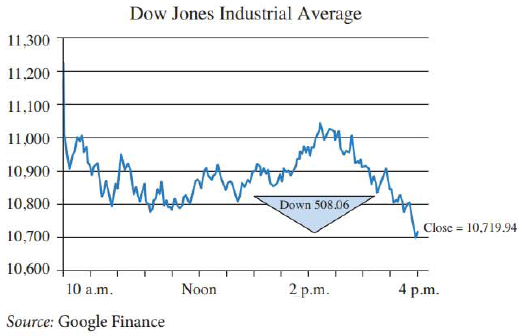Chapter 9.1, Problem 67E### Mathematical Applications for the ...

11th Edition
Ronald J. Harshbarger + 1 other
ISBN: 9781305108042

#### Solutions

Chapter
Section### Mathematical Applications for the ...

11th Edition
Ronald J. Harshbarger + 1 other
ISBN: 9781305108042
Textbook Problem

# Dow Jones Industrial Average The graph in the figure shows the Dow Jones Industrial Average (DJIA) on a particularly tumultuous day. Use the graph for Problems 69 and 70, with t as the time of day and D(t) as the DJIA at time t.Estimate lim t → 9 : 30 AM + D ( t ) , if it exists. Explain what this limit corresponds to.To determine

The value of the limit, limt9:30AM+D(t) if it exists and what it corresponds to.

Explanation

Given Information:

The graph shows the Dow Jones Industrial Average (DJIA) on a particularly tumultuous day.

Explanation:

Consider the provided graph,

The value of limt9:30AM+D(t) is the value of the graph when the values of x-coordinates approach 9:30a.m. with x>9:30

### Still sussing out bartleby?

Check out a sample textbook solution.

See a sample solution

#### The Solution to Your Study Problems

Bartleby provides explanations to thousands of textbook problems written by our experts, many with advanced degrees!

Get Started

#### Evaluate the expression sin Exercises 116. 22212424

Finite Mathematics and Applied Calculus (MindTap Course List)

#### If N{A}=31,N{B}=47,N{AB}=17,findN{AB}.

Elementary Geometry For College Students, 7e

#### The harmonic series is: 1 + 2 + 3 + 4 + …

Study Guide for Stewart's Single Variable Calculus: Early Transcendentals, 8th